Ex 3.1

Chapter 3 Class 10 Pair of Linear Equations in Two Variables (Term 1)
Serial order wise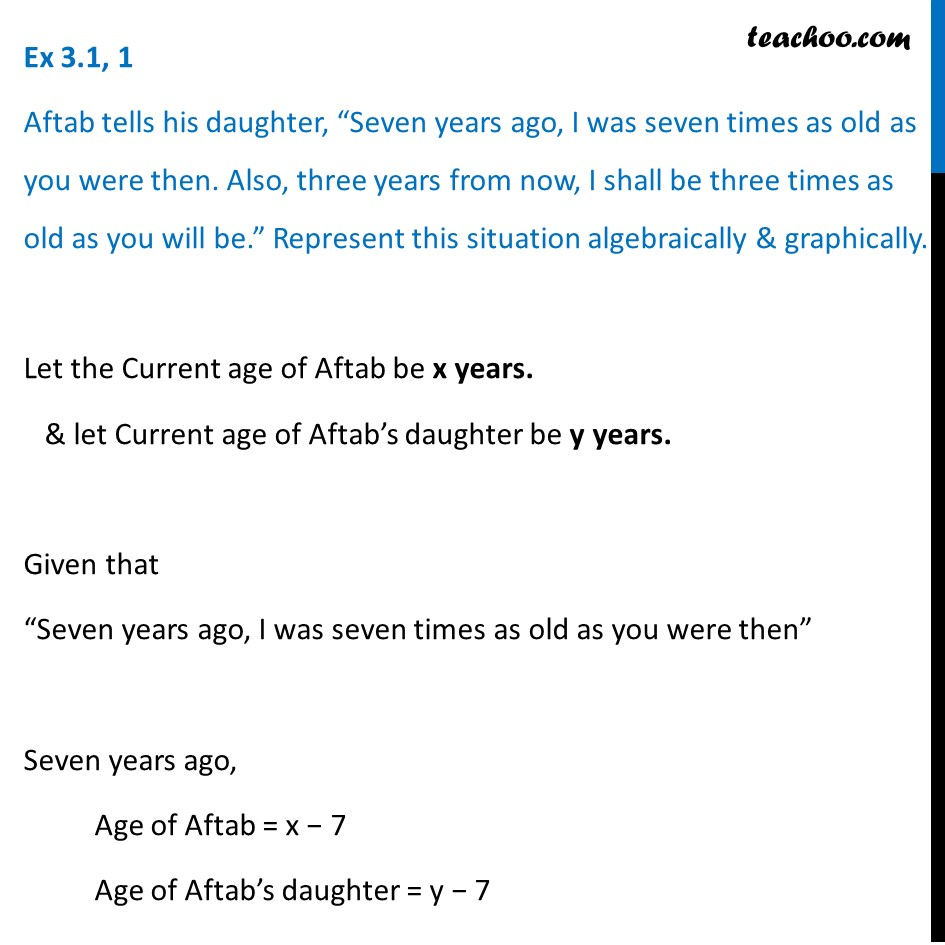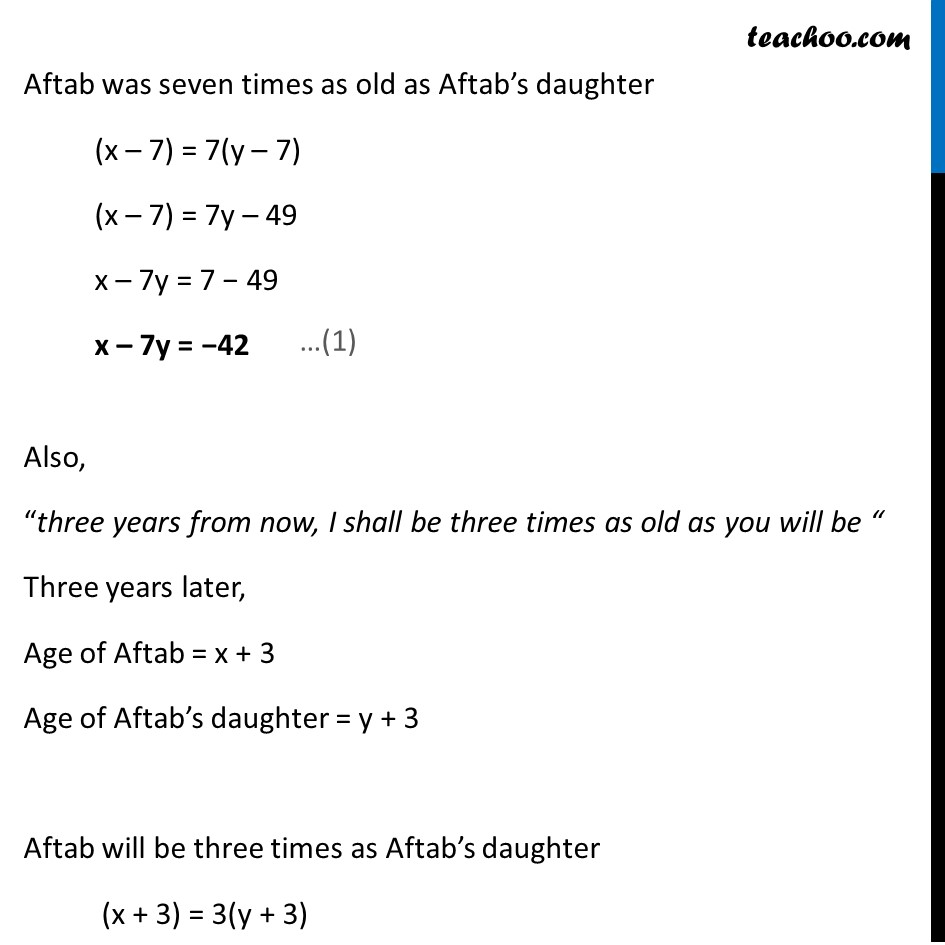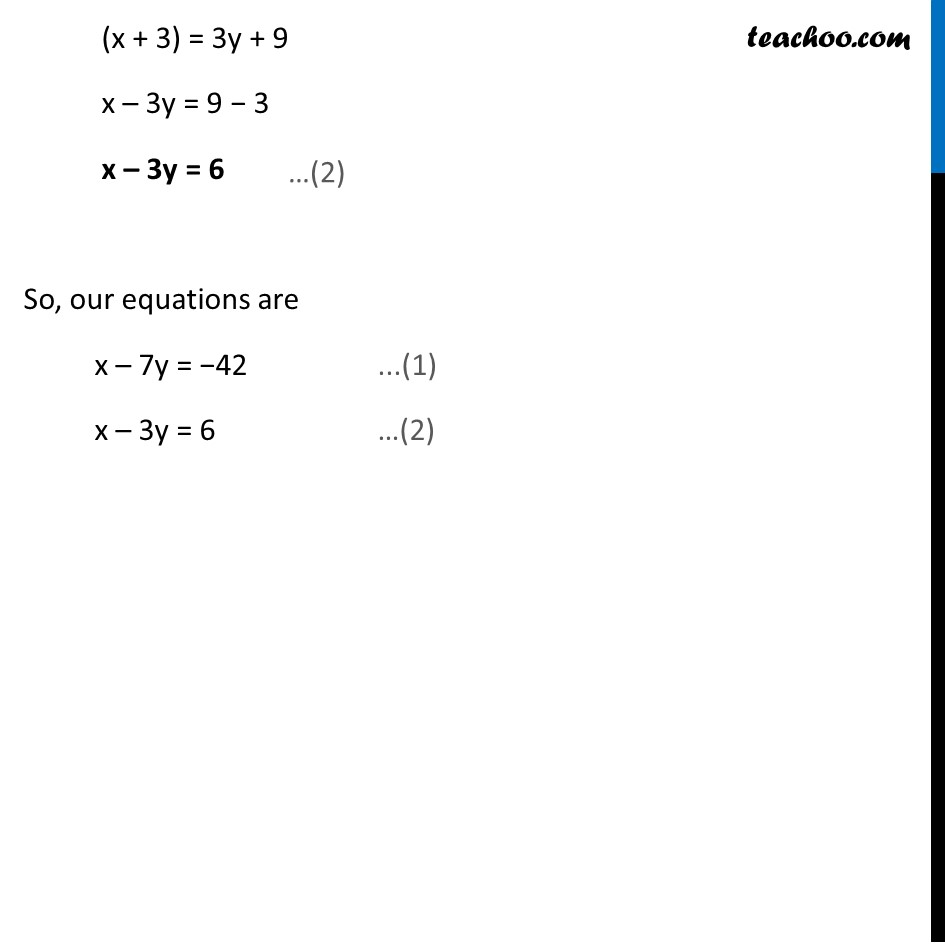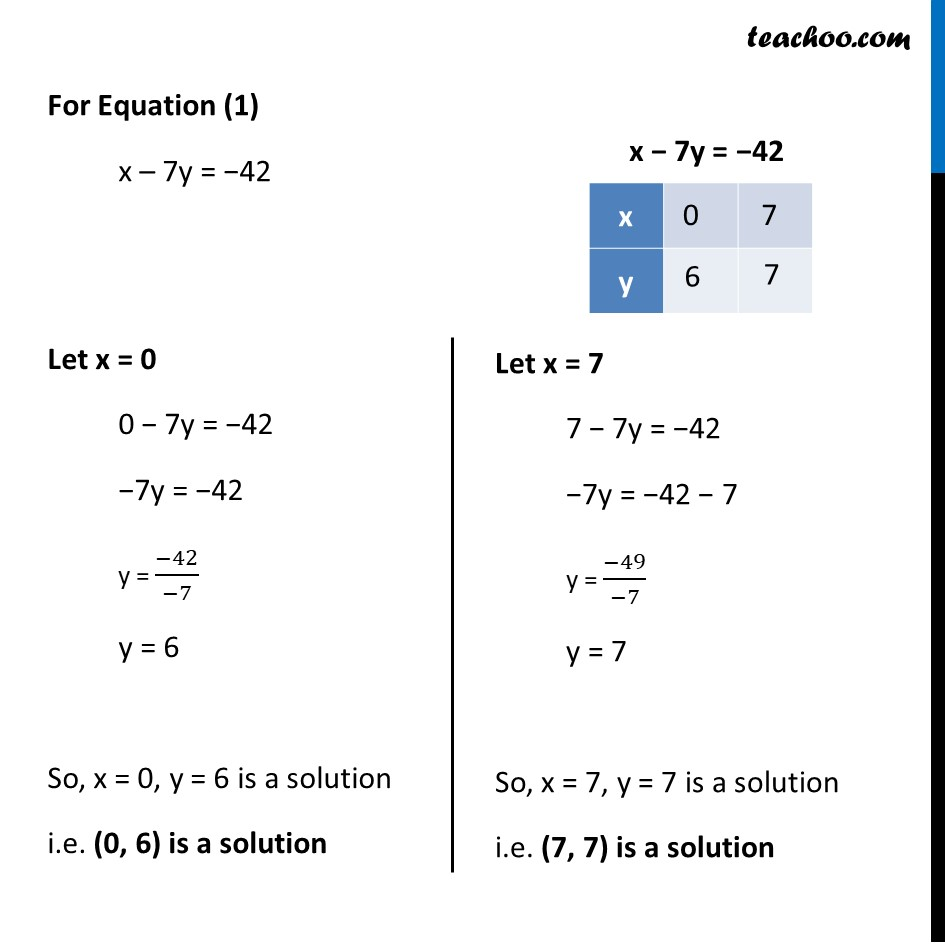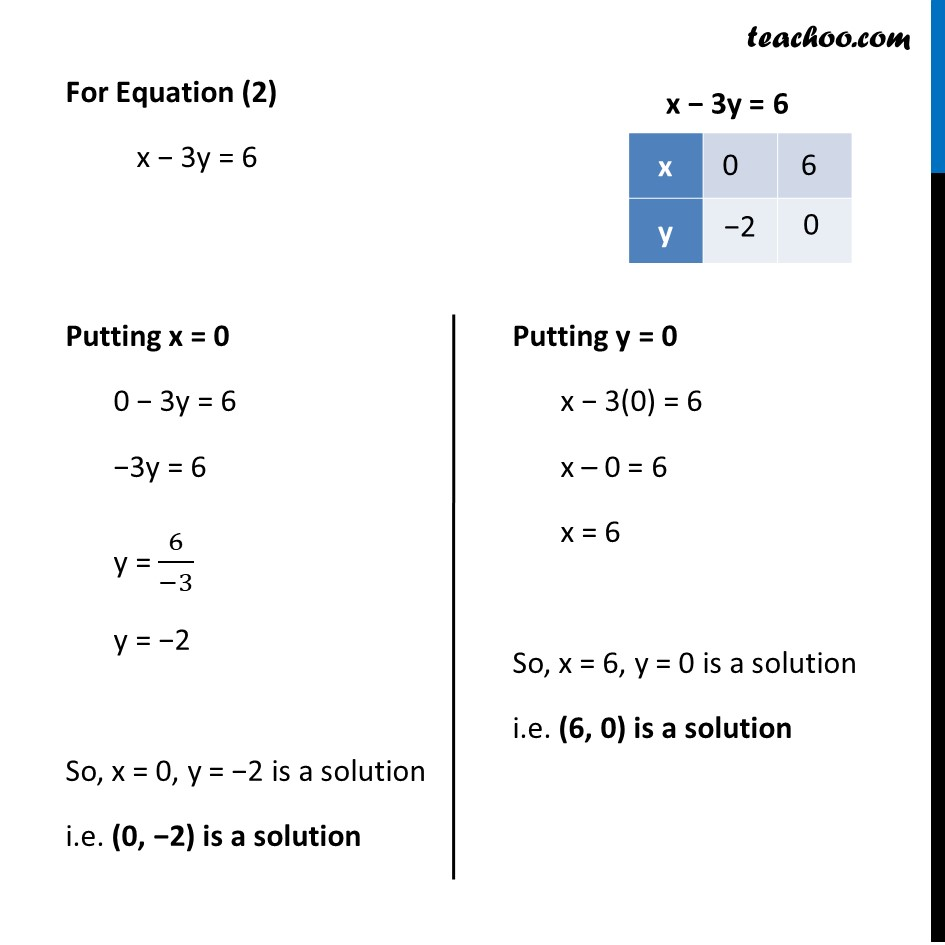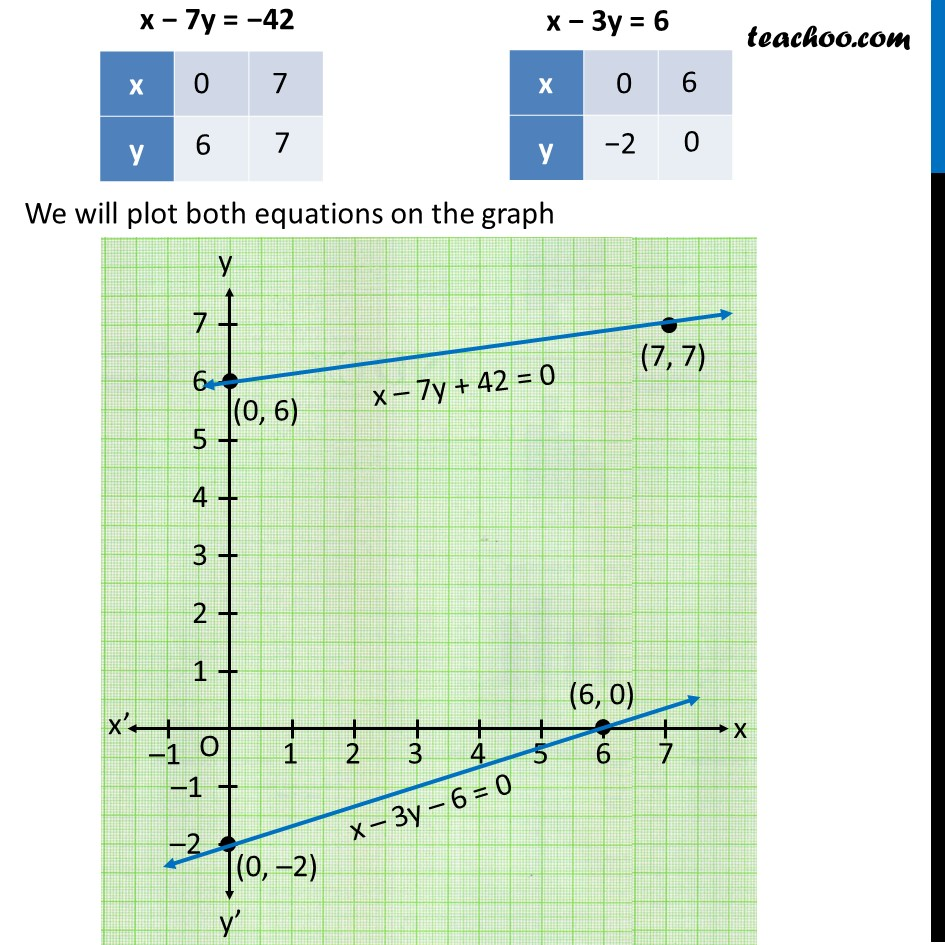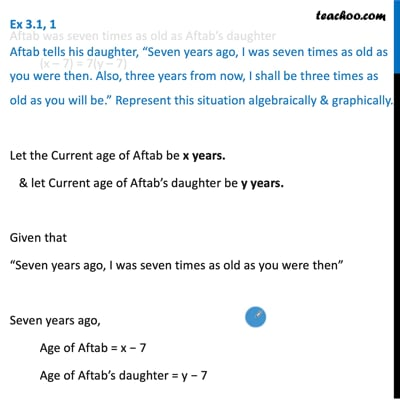This video is only available for Teachoo black users

### Transcript

Ex 3.1, 1 Aftab tells his daughter, “Seven years ago, I was seven times as old as you were then. Also, three years from now, I shall be three times as old as you will be.” Represent this situation algebraically & graphically. Let the Current age of Aftab be x years. & let Current age of Aftab’s daughter be y years. Given that “Seven years ago, I was seven times as old as you were then” Seven years ago, Age of Aftab = x − 7 Age of Aftab’s daughter = y − 7 Aftab was seven times as old as Aftab’s daughter (x – 7) = 7(y – 7) (x – 7) = 7y – 49 x – 7y = 7 − 49 x – 7y = −42 Also, “three years from now, I shall be three times as old as you will be “ Three years later, Age of Aftab = x + 3 Age of Aftab’s daughter = y + 3 Aftab will be three times as Aftab’s daughter (x + 3) = 3(y + 3) (x + 3) = 3y + 9 x – 3y = 9 − 3 x – 3y = 6 So, our equations are x – 7y = −42 ...(1) x – 3y = 6 For Equation (1) x – 7y = −42 Let x = 0 0 − 7y = −42 −7y = −42 y = (−42)/(−7) y = 6 So, x = 0, y = 6 is a solution i.e. (0, 6) is a solution Let x = 7 7 − 7y = −42 −7y = −42 − 7 y = (−49)/(−7) y = 7 So, x = 7, y = 7 is a solution i.e. (7, 7) is a solution For Equation (2) x − 3y = 6 Putting x = 0 0 − 3y = 6 −3y = 6 y = 6/(−3) y = −2 So, x = 0, y = −2 is a solution i.e. (0, −2) is a solution Putting y = 0 x − 3(0) = 6 x – 0 = 6 x = 6 So, x = 6, y = 0 is a solution i.e. (6, 0) is a solution We will plot both equations on the graph